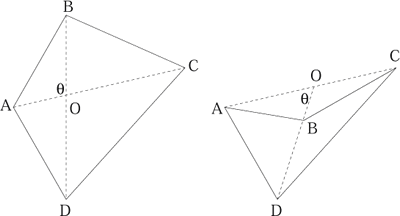#### You may also like### Degree Ceremony

What does Pythagoras' Theorem tell you about these angles: 90°, (45+x)° and (45-x)° in a triangle?### Logosquares

Ten squares form regular rings either with adjacent or opposite vertices touching. Calculate the inner and outer radii of the rings that surround the squares.### Ball Bearings

If a is the radius of the axle, b the radius of each ball-bearing, and c the radius of the hub, why does the number of ball bearings n determine the ratio c/a? Find a formula for c/a in terms of n.

# Diagonals for Area

##### Age 16 to 18 Challenge Level:

Tom and James from Queen Mary's Grammar School, Walsall and Shu Cao from Oxford High School gave this solution for a convex quadrilateral. Can you see how to adapt the solution for the case of the arrow shaped quadrilateral?Suppose there is a convex quadrilateral $ABCD$, the diagonals $AC$ and $BD$ cross each other at $O$. The angle between $AO$ and $BO$ is $\theta$ degrees, the angle between $DO$ and $CO$ is the same. The angle between $AO$ and $DO$ is $180-\theta$ degrees, the angle between $BO$ and $CO$ is the same.

The area of triangle $AOB$ is ${1\over 2}AO\times BO \sin \theta$.
The area of triangle $AOD$ is ${1\over 2}AO\times DO \sin (180-\theta)={1\over 2}AO\times DO \sin \theta$.
The area of triangle $DOC$ is ${1\over 2}DO\times CO \sin \theta$.
The area of triangle $BOC$ is ${1\over 2}BO\times CO \sin (180-\theta)={1\over 2}BO\times CO \sin \theta$.
The area of the quadrilateral is the sum of these four triangles.

\eqalign{ {\rm Area}&={1\over 2}AO\times BO \sin \theta+{1\over 2}AO\times DO \sin \theta+{1\over 2}DO\times CO \sin \theta+{1\over 2}BO\times CO \sin \theta \cr &= {1\over 2}[AO(BO+DO) + CO(DO+ BO)]\sin \theta \cr &={1\over 2}(AO\times BD+ CO\times BD)\sin \theta \cr &={1\over 2}AC\times BD \sin \theta }

So we have proved that for a convex quadrilateral the area of the quadrilateral is given by half the product of the lengths of the diagonals multiplied by the sine of the angle between the diagonals.